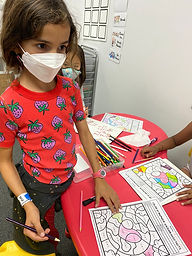## Ms. Hiral

### Target 1​

###### Lesson Type:

Continuation

Data Probability

:

Interpreting Data

Construct and read simple graphs from given information to solve problems.

###### 1:

Understand that a graph is a visual representation of data.

###### 2:

Become familiar with the vocabulary associated with graphs: unit, scale, title and labels.

###### 3:

Construct and read a scaled bar graph.

###### 4:

Understand how to use the scale to accurately read a bar graph.

3rd

###### Vocabulary:

Bar Graph, Unit, Scale, Title, Label

Activities:

Students played bowling, tallied up the scores, and created graphs using the data.

Students then compared their different graphs showing the same data and answered questions using their graphs.### Home Exploration

###### Guiding Questions:## Absent Students:

### Target 2

:

###### 1:

Understand that dividing numbers makes numbers smaller.

###### 2:

Understand that division represents taking a quantity and determining how many equal groups of objects can be made.

###### 3:

Understand there are multiple ways to divide a multi-digit number.

###### 4:

Understand that division equations can be written both vertically and horizontally and division can be indicated using multiple symbols (i.e., ÷, ⟌).

2nd

###### Vocabulary:

Divide, Equal Group, Symbol

Activities:

Students used base 10 blocks to practice division.

Students further practiced division with a color by numbers picture.### Home Exploration

###### Guiding Questions:### Target 3

:

###### 1:

Define perimeter.

###### 2:

Understand that perimeter is the distance around a figure.

###### 3:

Understand that perimeter can be found by adding the lengths of the sides of a figure.

###### 4:

See a link between finding the perimeter of rectangles and multiplication.

###### 5:

Understand that figures with the same perimeter can look different.

2nd

###### Vocabulary:

Perimeter, Distance

Activities:

Students practiced calculating perimeter with centimeter blocks and their workbooks.### Home Exploration